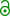## Indirect adjusted comparisons in BE [General Sta­tis­tics]

Dear all (and esp. Shuanghe),

I must confess that I don’t get what Luther Gwaza et al.1,2 means by his “pragmatic method”:

This method does not require the assumption of homogeneity of variances ($$SE_d=SE_{A}^{2}+SE_{B}^{2}$$), since it is un­likely verifiable, between studies with small sample sizes that follow Student’s t-test distribution (t0.9,d.f.), whose degrees of freedom are approximated for simplicity as if the variances were homogeneous (n1+n2–2).

Sounds great but how does he pool variances here? If they are weighted by the studies’ sample sizes – which IMHO, is the correct method – we end up in the homoscedastic method, where (in his notation):$$SD_{pooled}^{2}=\frac{(n_1-1)SD_{1}^{2}+(n_2-1)SD_{2}^{2}}{n_1+n_2-2}$$ BTW, is this a typo in the quote? A pooled variance as the sum? I suspect that he simply used the arithmetic mean which would not be correct even for equal samples & variances.
@Shuanghe: How did you do it?

1. Gwaza L, Gordon J, Welink J, Potthast H, Hansson H, Stahl M, García-Arieta A. Statistical approaches to indirectly compare bioequivalence between generics: a comparison of methodologies employing artemether / lume­fantrine 20/120 mg tablets as prequalified by WHO. Eur J Clin Pharmacol. 2012;68(12):1611–8. doi:10.1007/s00228-012-1396-1.
2. Gwaza L. Adjusted Indirect Treatment Comparisons of Bioequivalence Studies. PhD Thesis: Utrecht. 2016.free resource.

Cheers,
Helmut SchützThe quality of responses received is directly proportional to the quality of the question asked. 🚮
Science QuotesIng. Helmut Schütz